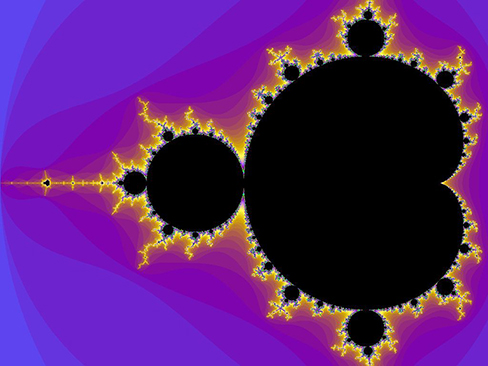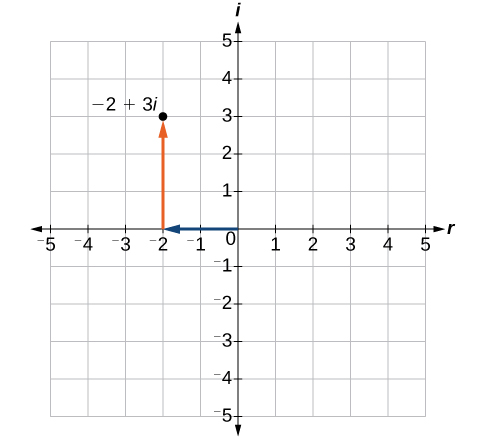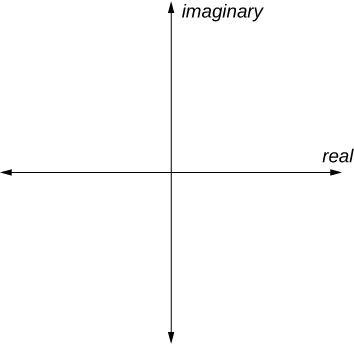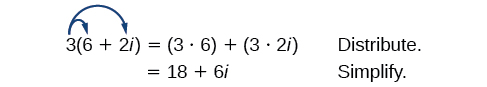## Complex Numbers

### Learning Objectives

In this section you will:

• Add and subtract complex numbers.
• Multiply and divide complex numbers.
• Simplify powers of $i$.Figure 1.

Discovered by Benoit Mandelbrot around 1980, the Mandelbrot Set is one of the most recognizable fractal images. The image is built on the theory of self-similarity and the operation of iteration. Zooming in on a fractal image brings many surprises, particularly in the high level of repetition of detail that appears as magnification increases. The equation that generates this image turns out to be rather simple.

In order to better understand it, we need to become familiar with a new set of numbers. Keep in mind that the study of mathematics continuously builds upon itself. Negative integers, for example, fill a void left by the set of positive integers. The set of rational numbers, in turn, fills a void left by the set of integers. The set of real numbers fills a void left by the set of rational numbers. Not surprisingly, the set of real numbers has voids as well. In this section, we will explore a set of numbers that fills voids in the set of real numbers and find out how to work within it.

### Expressing Square Roots of Negative Numbers as Multiples of$\,i$

We know how to find the square root of any positive real number. In a similar way, we can find the square root of any negative number. The difference is that the root is not real. If the value in the radicand is negative, the root is said to be an imaginary number. The imaginary number$\,i\,$is defined as the square root of$\,-1.$

$\sqrt{-1}=i$

${i}^{2}={\left(\sqrt{-1}\right)}^{2}=-1$

We can write the square root of any negative number as a multiple of$\,i.\,$Consider the square root of $\,-49.$

$\begin{array}{ccc}\hfill \sqrt{-49}& =& \hfill \sqrt{49\cdot \left(-1\right)}\\ & =& \sqrt{49}\sqrt{-1}\hfill \\ & =& 7i\hfill \end{array}$

We use$\,7i\,$and not$\,-7i\,$because the principal root of$\,49\,$is the positive root.

A complex number is the sum of a real number and an imaginary number. A complex number is expressed in standard form when written$\,a+bi\,$where$\,a\,$is the real part and$\,b\,$is the imaginary part. For example,$\,5+2i\,$is a complex number. So, too, is$\,3+4i\sqrt{3}.$Imaginary numbers differ from real numbers in that a squared imaginary number produces a negative real number. Recall that when a positive real number is squared, the result is a positive real number and when a negative real number is squared, the result is also a positive real number. Complex numbers consist of real and imaginary numbers.

### Imaginary and Complex Numbers

A complex number is a number of the form$\,a+bi\,$where

• $a\,$is the real part of the complex number.
• $b\,$is the imaginary part of the complex number.

If$\,b=0,$then$\,a+bi\,$is a real number. If$\,a=0\,$and$\,b\,$is not equal to 0, the complex number is called a pure imaginary number. An imaginary number is an even root of a negative number.

### How To

Given an imaginary number, express it in the standard form of a complex number.

1. Write$\,\sqrt{-a}\,$as$\,\sqrt{a}\sqrt{-1}.$
2. Express$\,\sqrt{-1}\,$as$\,i.\,$
3. Write$\,\sqrt{a}\cdot i\,$in simplest form.

### Expressing an Imaginary Number in Standard Form

Express$\,\sqrt{-9}\,$in standard form.

### Try It

Express$\,\sqrt{-24}\,$in standard form.

### Plotting a Complex Number on the Complex Plane

We cannot plot complex numbers on a number line as we might real numbers. However, we can still represent them graphically. To represent a complex number, we need to address the two components of the number. We use the complex plane, which is a coordinate system in which the horizontal axis represents the real component and the vertical axis represents the imaginary component. Complex numbers are the points on the plane, expressed as ordered pairs$\,\left(a,b\right),$where$\,a\,$represents the coordinate for the horizontal axis and$\,b\,$represents the coordinate for the vertical axis.

Let’s consider the number$\,-2+3i.\,$The real part of the complex number is$\,-2\,$and the imaginary part is 3. We plot the ordered pair$\,\left(-2,3\right)\,$to represent the complex number$\,-2+3i,$as shown in (Figure).Figure 2.

### Complex Plane

In the complex plane, the horizontal axis is the real axis, and the vertical axis is the imaginary axis, as shown in (Figure).Figure 3.

### How To

Given a complex number, represent its components on the complex plane.

1. Determine the real part and the imaginary part of the complex number.
2. Move along the horizontal axis to show the real part of the number.
3. Move parallel to the vertical axis to show the imaginary part of the number.
4. Plot the point.

### Plotting a Complex Number on the Complex Plane

Plot the complex number$\,3-4i\,$on the complex plane.

### Try It

Plot the complex number$\,-4-i\,$on the complex plane.

### Adding and Subtracting Complex Numbers

Just as with real numbers, we can perform arithmetic operations on complex numbers. To add or subtract complex numbers, we combine the real parts and then combine the imaginary parts.

### Complex Numbers: Addition and Subtraction

$\left(a+bi\right)+\left(c+di\right)=\left(a+c\right)+\left(b+d\right)i$

Subtracting complex numbers:

$\left(a+bi\right)-\left(c+di\right)=\left(a-c\right)+\left(b-d\right)i$

### How To

Given two complex numbers, find the sum or difference.

1. Identify the real and imaginary parts of each number.
2. Add or subtract the real parts.
3. Add or subtract the imaginary parts.

### Adding and Subtracting Complex Numbers

1. $\left(3-4i\right)+\left(2+5i\right)$
2. $\left(-5+7i\right)-\left(-11+2i\right)$

### Try It

Subtract$\,2+5i\,$from$\,3–4i.$

### Multiplying Complex Numbers

Multiplying complex numbers is much like multiplying binomials. The major difference is that we work with the real and imaginary parts separately.

#### Multiplying a Complex Number by a Real Number

Lets begin by multiplying a complex number by a real number. We distribute the real number just as we would with a binomial. Consider, for example,$\,3\left(6+2i\right)$:### How To

Given a complex number and a real number, multiply to find the product.

1. Use the distributive property.
2. Simplify.

### Multiplying a Complex Number by a Real Number

Find the product$\,4\left(2+5i\right).$

### Try It

Find the product:$\,\frac{1}{2}\left(5-2i\right).$

#### Multiplying Complex Numbers Together

Now, let’s multiply two complex numbers. We can use either the distributive property or more specifically the FOIL method because we are dealing with binomials. Recall that FOIL is an acronym for multiplying First, Inner, Outer, and Last terms together. The difference with complex numbers is that when we get a squared term,$\,{i}^{2},$it equals$\,-1.$

$\begin{array}{cccc}\hfill \left(a+bi\right)\left(c+di\right)& =& ac+adi+bci+bd{i}^{2}\hfill & \\ & =& ac+adi+bci-bd\hfill & \phantom{\rule{2em}{0ex}}{i}^{2}=-1\hfill \\ & =& \left(ac-bd\right)+\left(ad+bc\right)i\hfill & \phantom{\rule{2em}{0ex}}\text{Group real terms and imaginary terms}.\hfill \end{array}$

### How To

Given two complex numbers, multiply to find the product.

1. Use the distributive property or the FOIL method.
2. Remember that$\,{i}^{2}=-1.$
3. Group together the real terms and the imaginary terms

### Multiplying a Complex Number by a Complex Number

Multiply: $\,\left(4+3i\right)\left(2-5i\right).$

### Try It

Multiply:$\,\left(3-4i\right)\left(2+3i\right).$

### Dividing Complex Numbers

Dividing two complex numbers is more complicated than adding, subtracting, or multiplying because we cannot divide by an imaginary number, meaning that any fraction must have a real-number denominator to write the answer in standard form$\,a+bi.\,$We need to find a term by which we can multiply the numerator and the denominator that will eliminate the imaginary portion of the denominator so that we end up with a real number as the denominator. This term is called the complex conjugate of the denominator, which is found by changing the sign of the imaginary part of the complex number. In other words, the complex conjugate of$\,a+bi\,$is$\,a-bi.\,$For example, the product of$\,a+bi\,$and$\,a-bi\,$is

$\begin{array}{ccc}\hfill \left(a+bi\right)\left(a-bi\right)& =& {a}^{2}-abi+abi-{b}^{2}{i}^{2}\\ & =& {a}^{2}+{b}^{2}\hfill \end{array}$

The result is a real number.

Note that complex conjugates have an opposite relationship: The complex conjugate of$\,a+bi\,$is$\,a-bi,$and the complex conjugate of$\,a-bi\,$is$\,a+bi.\,$Further, when a quadratic equation with real coefficients has complex solutions, the solutions are always complex conjugates of one another.

Suppose we want to divide$\,c+di\,$by$\,a+bi,$where neither$\,a\,$nor$\,b\,$equals zero. We first write the division as a fraction, then find the complex conjugate of the denominator, and multiply.

$\frac{c+di}{a+bi}\text{ where }a\ne 0\text{ and }b\ne 0$

Multiply the numerator and denominator by the complex conjugate of the denominator.

$\frac{\left(c+di\right)}{\left(a+bi\right)}\cdot \frac{\left(a-bi\right)}{\left(a-bi\right)}=\frac{\left(c+di\right)\left(a-bi\right)}{\left(a+bi\right)\left(a-bi\right)}$

Apply the distributive property.

$=\frac{ca-cbi+adi-bd{i}^{2}}{{a}^{2}-abi+abi-{b}^{2}{i}^{2}}$

Simplify, remembering that$\,{i}^{2}=-1.$

$\begin{array}{l}=\frac{ca-cbi+adi-bd\left(-1\right)}{{a}^{2}-abi+abi-{b}^{2}\left(-1\right)}\hfill \\ =\frac{\left(ca+bd\right)+\left(ad-cb\right)i}{{a}^{2}+{b}^{2}}\hfill \end{array}$

### The Complex Conjugate

The complex conjugate of a complex number$\,a+bi\,$is$\,a-bi.\,$It is found by changing the sign of the imaginary part of the complex number. The real part of the number is left unchanged.

• When a complex number is multiplied by its complex conjugate, the result is a real number.
• When a complex number is added to its complex conjugate, the result is a real number.

### Finding Complex Conjugates

Find the complex conjugate of each number.

1. $2+i\sqrt{5}$
2. $-\frac{1}{2}i$

#### Analysis

Although we have seen that we can find the complex conjugate of an imaginary number, in practice we generally find the complex conjugates of only complex numbers with both a real and an imaginary component. To obtain a real number from an imaginary number, we can simply multiply by$\,i.$

### Try It

Find the complex conjugate of$\,-3+4i.$

### How To

Given two complex numbers, divide one by the other.

1. Write the division problem as a fraction.
2. Determine the complex conjugate of the denominator.
3. Multiply the numerator and denominator of the fraction by the complex conjugate of the denominator.
4. Simplify.

### Dividing Complex Numbers

Divide:$\,\left(2+5i\right)\,$by$\,\left(4-i\right).$

### Simplifying Powers of i

The powers of$\,i\,$are cyclic. Let’s look at what happens when we raise$\,i\,$to increasing powers.

$\begin{array}{l}{i}^{1}=i\hfill \\ {i}^{2}=-1\hfill \\ {i}^{3}={i}^{2}\cdot i=-1\cdot i=-i\hfill \\ {i}^{4}={i}^{3}\cdot i=-i\cdot i=-{i}^{2}=-\left(-1\right)=1\hfill \\ {i}^{5}={i}^{4}\cdot i=1\cdot i=i\hfill \end{array}$

We can see that when we get to the fifth power of$\,i,$it is equal to the first power. As we continue to multiply$\,i\,$ by increasing powers, we will see a cycle of four. Let’s examine the next four powers of$\,i.$

$\begin{array}{l}{i}^{6}={i}^{5}\cdot i=i\cdot i={i}^{2}=-1\hfill \\ {i}^{7}={i}^{6}\cdot i={i}^{2}\cdot i={i}^{3}=-i\hfill \\ {i}^{8}={i}^{7}\cdot i={i}^{3}\cdot i={i}^{4}=1\hfill \\ {i}^{9}={i}^{8}\cdot i={i}^{4}\cdot i={i}^{5}=i\hfill \end{array}$

The cycle is repeated continuously:$\,i,-1,-i,1,$every four powers.

### Simplifying Powers of$\,i$

Evaluate:$\,{i}^{35}.$

### Try It

Evaluate:$\,{i}^{18}$

Can we write$\,{i}^{35}\,$in other helpful ways?

As we saw in (Figure), we reduced$\,{i}^{35}\,$to$\,{i}^{3}\,$by dividing the exponent by 4 and using the remainder to find the simplified form. But perhaps another factorization of$\,{i}^{35}\,$may be more useful. (Figure) shows some other possible factorizations.

 Factorization of$\,{i}^{35}$ ${i}^{34}\cdot i$ ${i}^{33}\cdot {i}^{2}$ ${i}^{31}\cdot {i}^{4}$ ${i}^{19}\cdot {i}^{16}$ Reduced form ${\left({i}^{2}\right)}^{17}\cdot i$ ${i}^{33}\cdot \left(-1\right)$ ${i}^{31}\cdot 1$ ${i}^{19}\cdot {\left({i}^{4}\right)}^{4}$ Simplified form ${\left(-1\right)}^{17}\cdot i$ $-{i}^{33}$ ${i}^{31}$ ${i}^{19}$

Each of these will eventually result in the answer we obtained above but may require several more steps than our earlier method.

Access these online resources for additional instruction and practice with complex numbers.

### Key Concepts

• The square root of any negative number can be written as a multiple of$\,i.\,$See (Figure).
• To plot a complex number, we use two number lines, crossed to form the complex plane. The horizontal axis is the real axis, and the vertical axis is the imaginary axis. See (Figure).
• Complex numbers can be added and subtracted by combining the real parts and combining the imaginary parts. See (Figure).
• Complex numbers can be multiplied and divided.
• To multiply complex numbers, distribute just as with polynomials. See (Figure) and (Figure).
• To divide complex numbers, multiply both numerator and denominator by the complex conjugate of the denominator to eliminate the complex number from the denominator. See (Figure) and (Figure).
• The powers of$\,i\,$are cyclic, repeating every fourth one. See (Figure).

### Section Exercises

#### Verbal

Explain how to add complex numbers.

What is the basic principle in multiplication of complex numbers?

Give an example to show that the product of two imaginary numbers is not always imaginary.

What is a characteristic of the plot of a real number in the complex plane?

#### Algebraic

For the following exercises, evaluate the algebraic expressions.

If$\,y={x}^{2}+x-4,$evaluate$\,y\,$given$\,x=2i.$

If$\,y={x}^{3}-2,$evaluate$\,y\,$given$\,x=i.$

If$\,y={x}^{2}+3x+5,$evaluate$\,y\,$given$\,x=2+i.$

If$\,y=2{x}^{2}+x-3,$evaluate$\,y\,$given$\,x=2-3i.$

If$\,y=\frac{x+1}{2-x},$evaluate$\,y\,$given$\,x=5i.$

If$\,y=\frac{1+2x}{x+3},$evaluate$\,y\,$given$\,x=4i.$

#### Graphical

For the following exercises, plot the complex numbers on the complex plane.

$1-2i$

$-2+3i$

$i$

$-3-4i$

#### Numeric

For the following exercises, perform the indicated operation and express the result as a simplified complex number.

$\left(3+2i\right)+\left(5-3i\right)$

$\left(-2-4i\right)+\left(1+6i\right)$

$\left(-5+3i\right)-\left(6-i\right)$

$\left(2-3i\right)-\left(3+2i\right)$

$\left(-4+4i\right)-\left(-6+9i\right)$

$\left(2+3i\right)\left(4i\right)$

$\left(5-2i\right)\left(3i\right)$

$\left(6-2i\right)\left(5\right)$

$\left(-2+4i\right)\left(8\right)$

$\left(2+3i\right)\left(4-i\right)$

$\left(-1+2i\right)\left(-2+3i\right)$

$\left(4-2i\right)\left(4+2i\right)$

$\left(3+4i\right)\left(3-4i\right)$

$\frac{3+4i}{2}$

$\frac{6-2i}{3}$

$\frac{-5+3i}{2i}$

$\frac{6+4i}{i}$

$\frac{2-3i}{4+3i}$

$\frac{3+4i}{2-i}$

$\frac{2+3i}{2-3i}$

$\sqrt{-9}+3\sqrt{-16}$

$-\sqrt{-4}-4\sqrt{-25}$

$\frac{2+\sqrt{-12}}{2}$

$\frac{4+\sqrt{-20}}{2}$

${i}^{8}$

${i}^{15}$

${i}^{22}$

#### Technology

For the following exercises, use a calculator to help answer the questions.

Evaluate$\,{\left(1+i\right)}^{k}\,$for$\,k=4,8,\text{and}\,12.\,$Predict the value if$\,k=16.$

Evaluate$\,{\left(1-i\right)}^{k}\,$for$\,k=2,6,\text{and}\,10.\,$Predict the value if$\,k=14.$

Evaluate${\left(\text{l}+i\right)}^{k}-{\left(\text{l}-i\right)}^{k}$for$\,k=4,8,\text{and}\,12.\,$Predict the value for$\,k=16.$

Show that a solution of$\,{x}^{6}+1=0\,$is$\,\frac{\sqrt{3}}{2}+\frac{1}{2}i.$

Show that a solution of$\,{x}^{8}-1=0\,$is$\,\frac{\sqrt{2}}{2}+\frac{\sqrt{2}}{2}i.$

#### Extensions

For the following exercises, evaluate the expressions, writing the result as a simplified complex number.

$\frac{1}{i}+\frac{4}{{i}^{3}}$

$\frac{1}{{i}^{11}}-\frac{1}{{i}^{21}}$

${i}^{7}\left(1+{i}^{2}\right)$

${i}^{-3}+5{i}^{7}$

$\frac{\left(2+i\right)\left(4-2i\right)}{\left(1+i\right)}$

$\frac{\left(1+3i\right)\left(2-4i\right)}{\left(1+2i\right)}$

$\frac{{\left(3+i\right)}^{2}}{{\left(1+2i\right)}^{2}}$

$\frac{3+2i}{2+i}+\left(4+3i\right)$

$\frac{4+i}{i}+\frac{3-4i}{1-i}$

$\frac{3+2i}{1+2i}-\frac{2-3i}{3+i}$

### Glossary

complex conjugate
a complex number containing the same terms as another complex number, but with the opposite operator. Multiplying a complex number by its conjugate yields a real number.
complex number
the sum of a real number and an imaginary number; the standard form is$\,a+bi,$where a is the real part and$\,b\,$is the complex part.
complex plane
the coordinate plane in which the horizontal axis represents the real component of a complex number, and the vertical axis represents the imaginary component, labeled i.
imaginary number
the square root of$\,-1\,$:$\,i=\sqrt{-1}.$